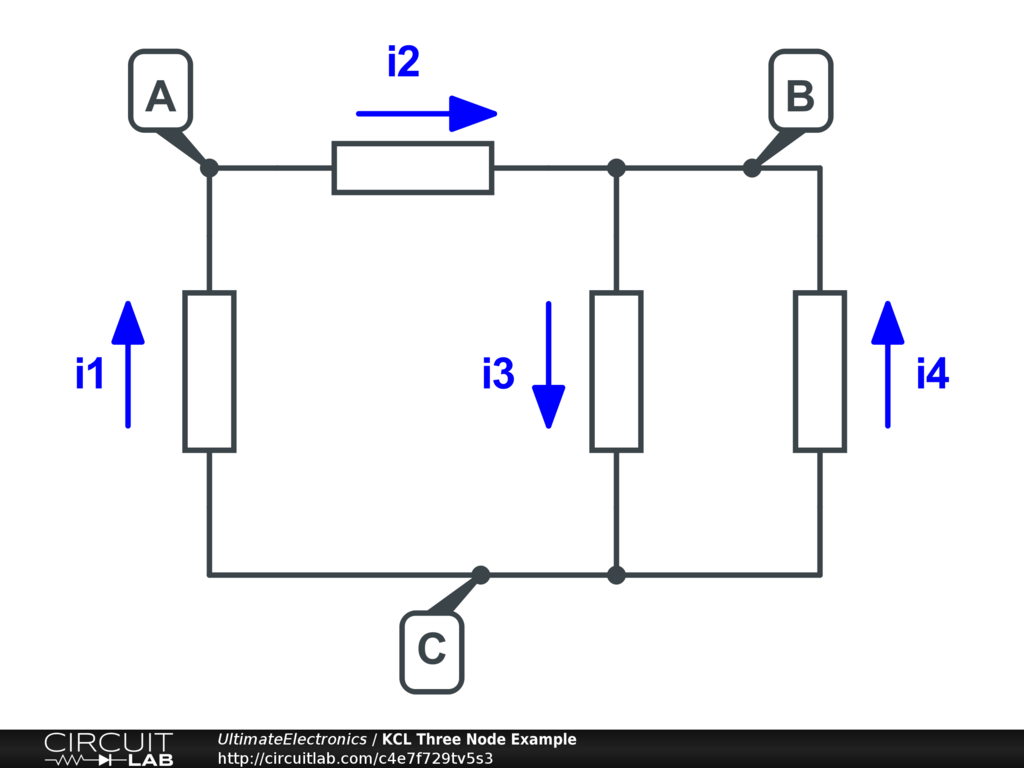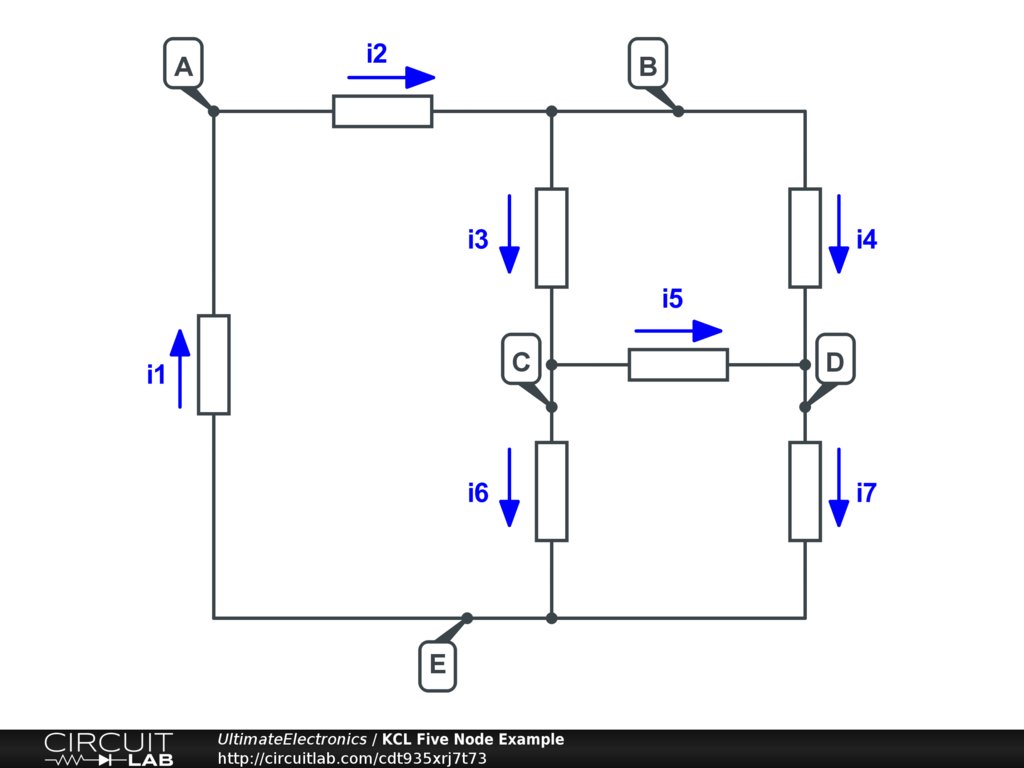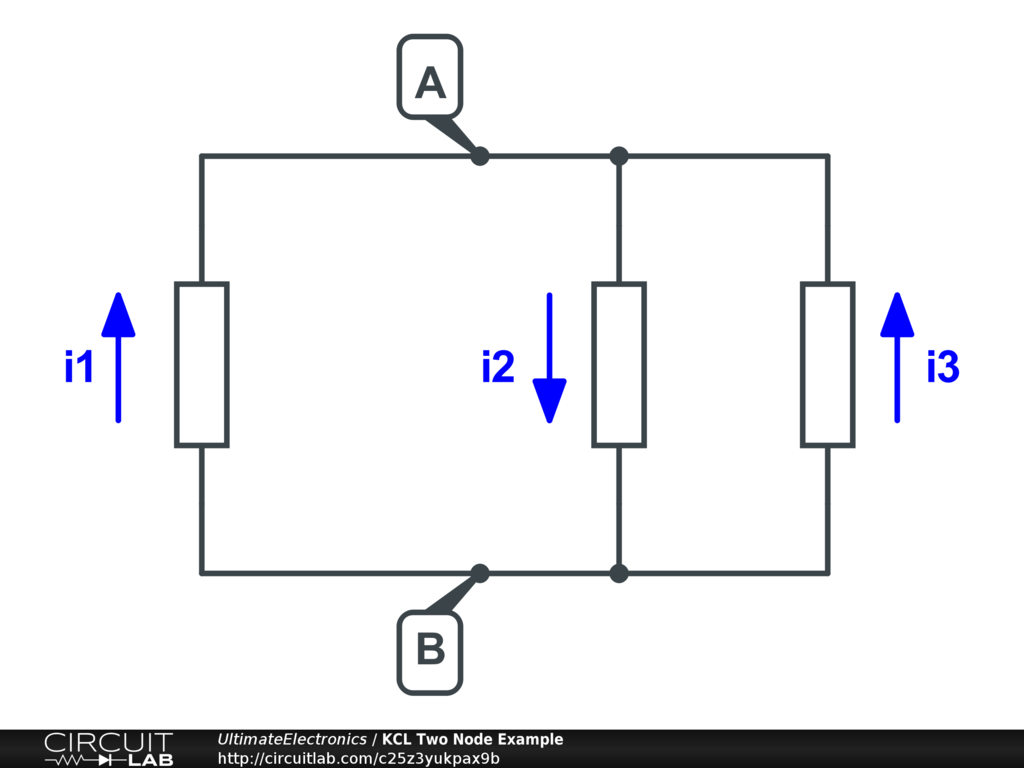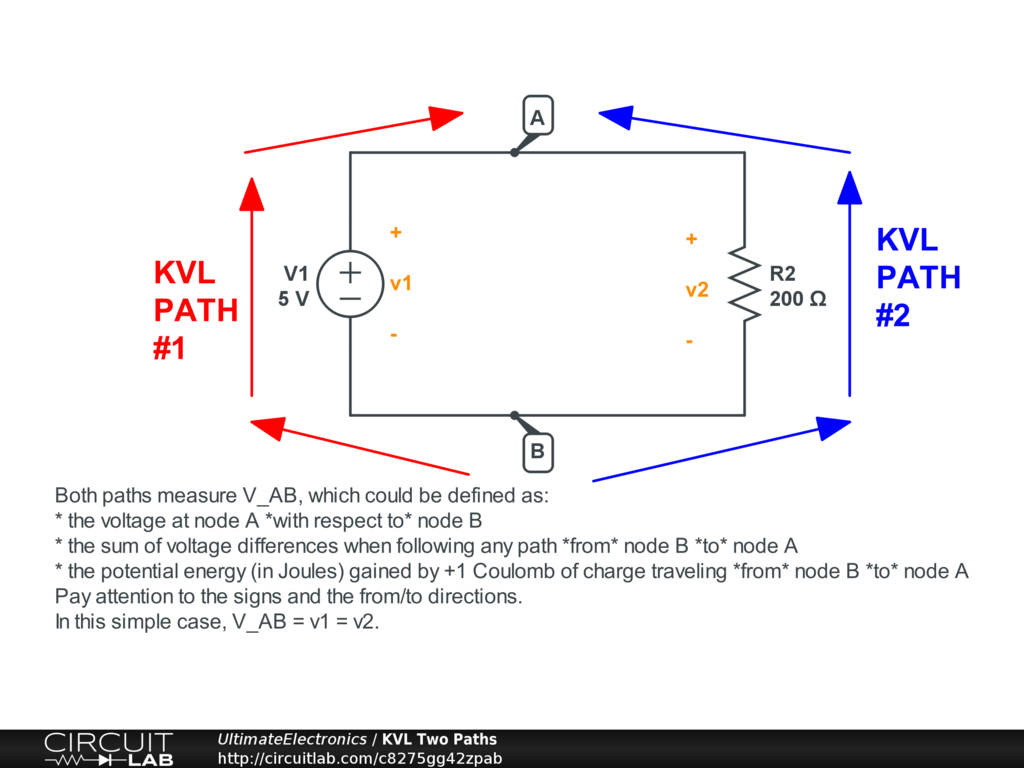Kirchhoff's Voltage Law and Kirchhoff's Current Law

In the previous section on Resistors in Series & Parallel, we built up a lot of intuition about how to think about current & voltage in a circuit. (If you haven't read that section, please go back and do so now.)

The resistor-specific combination rules for resistors in series and parallel will not generalize to other circuit elements. However, there are two basic principes that will generalize:

1. Two components in series will have the same current through them. We'll make this statement a bit broader and it will become Kirchhoff's Current Law.
2. Two components in parallel will have the same voltage across them. We'll make this statement a bit broader and it will become Kirchhoff's Voltage Law.

These two Kirchhoff's Laws will then become our basis for how to write the equations that describe how current and voltage behave in any electronic circuit.

In this section we're only concerned with how to write these equations. In other sections, including the Systems of Equations section from the previous chapter, we'll discuss how to solve those equations once written.

Kirchhoff's Current Law (KCL)

Kirchhoff's Current Law is a statement of conservation of charge: what goes in must come out at every junction (node) on a circuit network.

Under the Lumped Element Model, charge can't be stored at any node on the schematic, so if charge flows out of one element at node A, the same amount of current must instantaneously flow into a connected element's terminal at node A.

As intuition for why this must be true, remember that electrons can't enter or leave the system anywhere (there are no "leaks"), and electrons can't "pile up" anywhere because they'd repel each other.

This is just like the hydraulic analogy with the flow of water in a pipe network: at any pipe junction there are 2 or more connections, and any water that goes in must go out!

The direction of current is important too: we have to define currents with arrows going in or out and label them carefully. (We'll discuss this more in the next section, Labeling Voltages, Currents, and Nodes.)

Consider this circuit network with three nodes and four elements:In the circuit above, we have three nodes. We can write Kirchhoff's Current Law at each of the three nodes.

Mathematically, one way of writing it at each node is:

$$\sum i = 0$$

This says that all the currents at a node sum to zero.

We have to keep track and use a positive sign if the current flows into the node, and a negative sign if the current flows out.

In the example above, going through each node, the KCL equations are:

\begin{align} i_1 - i_2 & = 0 &&\text{Node A}\\ i_2 - i_3 + i_4 & = 0 &&\text{Node B}\\ -i_1 + i_3 - i_4 & = 0 &&\text{Node C} \end{align}

Another way of stating Kirchhoff's Current Law is:

$$\sum_{in} i = \sum_{out} i$$

In the example above the three equations would be:

\begin{align} i_1 & = i_2 &&\text{Node A}\\ i_2 + i_4 & = i_3 &&\text{Node B}\\ i_3 & = i_1 + i_4 &&\text{Node C} \end{align}

In this formulation, we are saying that the sum of the currents into a node equals the sum of the currents out of that node.

This is mathematically identical to the first way of stating the KCL, because those currents would just have negative sign.

Be sure to be careful of direction! It doesn't especially matter which direction you choose to label each current, but it is absolutely critical that it be consistent; current into one node is current out of another.

We can write the KCL at every node in a circuit. A node is just a place where elements connect.

Note that nodes may be bigger than they first appear: we can name nodes $A, B, C$ and refer to these names multiple places on a schematic, even if there aren't wires drawn explicitly between them. Additionally, the ground node is a special case of a named node, and is also connected together everywhere.

We can write the KCL equations without knowing anything about the components; it only specifies the topology (the shape) of how things connect to each other.

Here's a slightly more complicated example with 5 nodes and 7 edges. Notice that we label all the nodes, and then we label all the currents and their directions:Here are the KCL equations at each node that result when you sum all currents to zero:

\begin{align} i_1 - i_2 & = 0 &&\text{Node A} \\ i_2 - i_3 - i_4 & = 0 &&\text{Node B} \\ i_3 - i_5 - i_6 & = 0 &&\text{Node C} \\ i_4 + i_5 - i_7 & = 0 &&\text{Node D} \\ i_6 + i_7 - i_1 & = 0 &&\text{Node E} \end{align}

And, for completeness, here are the KCL equations at each node that result when you sum all currents in equal to all currents out:

\begin{align} i_1 & = i_2 &&\text{Node A} \\ i_2 & = i_3 + i_4 &&\text{Node B} \\ i_3 & = i_5 + i_6 &&\text{Node C} \\ i_4 + i_5 & = i_7 &&\text{Node D} \\ i_6 + i_7 & = i_1 &&\text{Node E} \end{align}

These two systems of equations are algebraically the same. Look closely at whichever one makes more sense to you, and observe how you can transform one into the other.

In the next section we'll talk about labeling currents so they're done consistenly with respect to direction and sign -- a common trap for beginners.

As we've hinted in Linear & Nonlinear and Systems of Equations, it is useful to develop some linear algebra intuition. The above series of KCL equations for the five-node example could be written as:

$$\begin{equation} \begin{bmatrix} 1 & -1 & 0 & 0 & 0 & 0 & 0 \\ 0 & 1 & -1 & -1 & 0 & 0 & 0 \\ 0 & 0 & 1 & 0 & -1 & -1 & 0 \\ 0 & 0 & 0 & 1 & 1 & 0 & -1 \\ -1 & 0 & 0 & 0 & 0 & 1 & 1 \\ \end{bmatrix} \begin{bmatrix} i_1 \\ i_2 \\ i_3 \\ i_4 \\ i_5 \\ i_6 \\ i_7 \end{bmatrix} = 0 \end{equation}$$

This alone isn't yet a solvable system of equations, however it contributes a big part to the overall system of equations that make up a solvable circuit.

Exceptions to Kirchhoff's Current Law (KCL)

There are no exceptions to Kirchhoff's Current Law -- by definition.

Note that while electrons within conductors would repel each other, for an insulator, the electrons might be "stuck" -- a static charge. It is possible for static charge to accumulate on and within a circuit; however, instead of considering the KCL to be broken, this effect is best modeled by adding capacitances at the nodes in question.

One Too Many Nodes

If we have $n$ nodes in our circuit, we can write $n$ KCL equations -- one at each node.

However, those equations will not be linearly independent. (For a review of linear independence and why it's critically important, review Systems of Equations.)

Consider this simple circuit with two nodes:We can write the KCL at node A:

$$i_1 - i_2 + i_3 = 0$$

And next we can write the KCL at node B:

$$-i_1 + i_2 - i_3 = 0$$

It should be obvious that these are, in fact, the same equation written twice; we've just multiplied one of them by -1.

Writing it down twice (once per node) didn't actually add any information. The second equation didn't add any new constraints that the first equation didn't already include.

That's because every edge in the graph adds a current term to one node's KCL equation, and subtracts that current term from another KCL equation. We're double counting the flows in and out everywhere even though all charge is conserved, which leads to this useless extra equation.

This extends to more complicated examples just as well. Consider again the three node example we looked at above:We can write the KCL at each node as we did above:

\begin{align} i_1 - i_2 & = 0 &&\text{Node A}\\ i_2 - i_3 + i_4 & = 0 &&\text{Node B}\\ -i_1 + i_3 - i_4 & = 0 &&\text{Node C} \end{align}

In this slightly more complicated case, it's less "obvious" that these aren't linearly independent, but it's still true. To see that, add equation #1 and equation #2, then multiply by -1, and you'll get equation #3.

This is a common trap for beginner problem solving, so keep an eye out for it.

In practice, the solution is to not write the KCL equation for the node picked to be the ground node. We'll talk more about that in the next section.

Kirchhoff's Voltage Law (KVL)

Kirchhoff's Voltage Law can be framed in a few different ways with the same underlying meaning.

We've already discussed in Voltage and Current how voltage is always a difference across two points. Even when we define a ground node for convenience, we're still looking at a voltage difference to that arbitrarily defined ground.

The first way of stating Kirchhoff's Voltage Law is that the total voltage difference between two points A and B is the same no matter what path you take.

This is like saying that the difference between a 5 foot tall person and a 6 foot tall person is always going to be 1 foot. It doesn't matter if we:

1. Put the two people back to back and measure the difference from the top of one head to another, or
2. Measure both from head to toe and do the subtraction, or
3. Measure them both from the ceiling and do the subtraction,
4. Have both people stand on a box and measure from the bottom of the box and do the subtraction.

In all four cases, we'll get 1 foot as the height difference.

Let's put these two people in a room and say that the x-y plane is the floor, and the z axis points toward the ceiling.

Now, imagine in your mind that all four of these ways of measuring are different paths in space between points A (first person's top-of-head) and B (second person's top-of-head). We're going to walk along the curve of each path and add up only the z-axis vertical distance -- keeping track of positive and negative -- as we walk along those four paths. We'll always get 1 foot as the vertical difference regardless of which path we take between A and B.

We can ignore the motion in the other directions because only the height difference matters. (And, in the same way for voltages, only the electric fields parallel to the path matter.)

It may sound simple, but that's really all there is to Kirchhoff's Voltage Law.

There's a second common way of stating the KVL: the sum of voltages around any loop is zero. A loop is defined as any path that starts and ends at the same point.

To apply to our height analogy, now it's saying that if you start from the top of the 5 foot tall person's head and make any loop in space, and you add up the changes in height (z-axis) as you go, you'll get zero when you return back to the starting point.

The "every loop sums up to zero" and the "every path between A and B has the same voltage difference" statements are mathematically identical, because you can always take a path from A to some point Q, and then add any path back from Q back to A to make a loop.

If you've studied multivariable calculus, this is a version of a line integral in a vector field -- in this case, the electric field -- and a potential function exists (the voltage itself), so the line integral is path independent, and the electric field is the gradient of the potential function. (We discussed this more in the Electrons at Rest section.)

We've just talked a bunch about measuring people's heights, but what's that got to do with electronics?

Well, just as height is a way of measuring the gravitational potential energy of a mass in a gravitational field, similarly voltage is a way of measuring the electric potential energy of charge in an electric field.

Let's say we have height A (higher) and height B (lower) and a bunch of little steel ball bearings. On the left, we've built a box that takes in balls from height B and raises them up to height A. On the right, the balls that come out from the box go down a high-friction ramp, where they roll down and eventually stop back at the bottom, at height B. From there, they go back into the box on the left to continue their cycle.

If we map the masses to charges, and map the heights to voltages, we've just described something like this very simple circuit with one voltage source and one resistor:Kirchhoff's Voltage Law says that the voltage difference between the two points we've labeled A and B is the same whether we take the path through the voltage source or the path through the resistor. Here are a few interchangeable definitions in mathematical terms:

$$v_{A B} = v_{\text{at}\ A\ \text{with respect to}\ B}$$

$$v_{A B} = \sum_{\text{any path from}\ B\ \text{to}\ A} v$$

$$v_{A B} = v_{B \to A\ \text{measured through voltage source}} = v_{B \to A\ \text{measured through resistor}}$$

$$v_{A B} = v_1 = v_2$$

Pay close attention to the signs and definitions of path directions. We'll explore these issues more closely in the next section.

The box on the left is like a voltage source: it takes the ball bearings (charges) and moves them from a lower potential energy state to a higher one.

The ramp on the right is like a resistor: it takes ball bearings (charges) from a high potential energy state back down to a lower one, dissipating that energy as heat along the way.

Kirchhoff's Voltage Law tells us that the potential energy (per unit charge) gained in going "up" the voltage source is equal to the potential energy (per unit charge) lost going "down" the resistor. That's the way of formulating that sentence as a loop: you see that the ball bearings (charges) make a complete loop.

We could make the loop version of the KVL by saying:

$$v_{B B} = 0$$ $$v_{B B} = v_{B \to A\ \text{measured through voltage source}} + v_{A \to B\ \text{measured through resistor}}$$ $$v_{B B} = v_{B \to A\ \text{measured through voltage source}} + (-v_{B \to A\ \text{measured through resistor}})$$ $$v_{B B} = v_1 + (-v_2)$$ $$0 = v_1 - v_2$$ $$v_1 = v_2$$

If it helps your understanding, another reason why Kirchoff's Voltage Law must be true is because of conservation of energy: if it weren't true, than a charge could follow a path around a circuit, flow through a few components, end up back where it started, and have gained potential energy! This would be absolutely great for perpetual motion machines, but not so great for the laws of thermodynamics.

Note that Kirchhoff's Voltage Law is determined in terms of summing up voltage differences. As discussed previously in Voltage and Current all voltages are relative, but sometimes we (for convenience) define a ground which is our $v=0$ reference. In our height measurement example, this is like saying that it doesn't matter whether we say $z=0$ at the floor, or $z=0$ at the shorter person's belly button. It's arbitrary. No matter what, the z-axis differences we add up will be the same.

Exceptions to Kirchhoff's Voltage Law

The fascinating thing about Kirchhoff's Voltage Law is that we've just argued so strongly for why it must be "obviously" true...

However, it may surprise you to learn that in the underlying physics of Maxwell's equations, the KVL is actually false! Faraday's law of induction is:

$$\oint \vec{E} \cdot \vec{d l} = -\frac {d \Phi_{B}} {dt}$$

This says that the voltage induced in a loop is equal to the rate of change of magnetic flux through the surface enclosed by the loop. So the voltage around a loop is zero only if there's no time-varying magnetic flux through that loop.

We referred to this problem in our discussion of Electrons at Rest. To summarize: our Lumped Element Model requires that we assume that Kirchhoff's Voltage Law holds, but we make some adjustments sometimes.

For example, every inductor and transformer generally has time-varying magnetic flux, but we just include these in the model of the circuit element itself. The voltage of an inductor is in fact the same as the right-hand term in Faraday's law, but instead of considering it an adjustment to the KVL, we consider it as a voltage source itself.

If there are external time-varying magnetic fields, however, we may have to worry about them. This can a source of interference in electronics. This is a reason why large electronic systems with loops inside can be a problem, and one of the reason why ground loops are a problem as well: they form a large surface for time-varying magnetic flux to cause spurious voltages in our system. However, we can usually model this effect as an additional voltage source if we like.

For now, though, you should assume that Kirchhoff's Voltage Law is true in your study of electronics. Just store this detail in the event you start working with time-varying magnetic fields at a later point!

A Brief Review

We're now at the point where we are starting to put together many of the pieces we've built in earlier sections:

• The Lumped Element Model and Thermodynamics, Energy & Equilibrium provide a high level conceptual framework for looking at systems including circuits.
• Systems of Equations provides the tools to know when and how we can solve many simultaneous constraints.
• Electrons at Rest gives us an understanding of electric forces, fields, and potentials (voltages).
• Electrons in Motion helps us think about how those forces get charges moving to create currents.
• Voltage and Current are the basic variables of potential energy and flow rate within electric circuits.
• Resistors in Series & Parallel gives us the intuition about how voltage and current behave when we combine multiple elements.
• And finally, Kirchhoff's Voltage Law and Kirchhoff's Current Law (this section) formalizes that intuition and allows us to describe the constraints on voltage and current within the architecture of any circuit network.

The next pieces of the puzzle are to combine the KCL and KVL equations with specific circuit element equations (like Ohm's Law), while carefully labeling all currents and voltages, and then solving those full systems of equations to understand how those constraints and components interact to produce a particular circuit's behavior.

What's Next

In the next section, Labeling Voltages, Currents, and Nodes, we'll discuss how to properly label the names and directions of all the voltages and currents within a circuit, which is essential for setting up a consistent set of circuit equations.